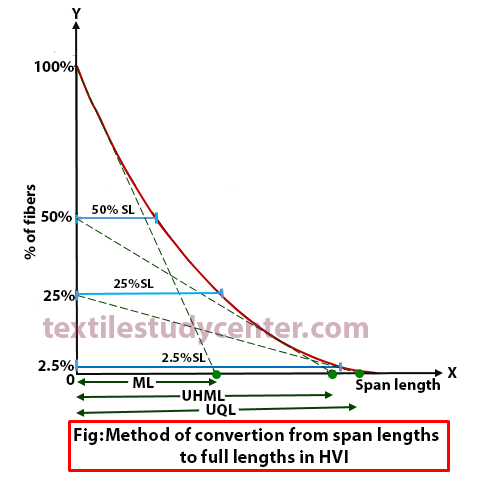# Span Length

10.15K
0#### Md Sohanur Rahman Sobuj

Co-founder , Admin & Author at Textile Study Center
Student of Bangladesh University of Textiles (BUTEX) Department: Apparel Engineering# Span Length

Fiber Length-1   Fiber Length-2  Fiber Length-3  Fiber Length-4  Fiber Length-5  Fiber Length-6

Span Length:

Span length is the distance exceeded by a stated percentage of fibers from a random catch point in drafting zone. 2.5% and 50% span length are the most commonly used by industry.

2.5% Span Length and 50% Span Length:

x % Span length is the distance spanned by x %of fibers in the specimen being tested when the fibers are parallelized and randomly distributed and where the initial starting point of the scanning in the test is considered 100%. This length is measured using “Digital Fibrograph‘.

Significance of 2.5% Span Length-

American dept. of agriculture shows that 2.5% span length best matches with the staple length assessed by the classer and hence 2.5%span has become a universal standard for evaluating cotton.

2.5% staple length is suitable for the spinning process.

The South India Textile Research Association (SITRA) gives the following empirical relationships to estimate the Effective Length and Mean Length from the Span lengths.

Uniformity Ratio:

The ratio between 50% span length and 2.5% span length is called uniformity ratio, express as a percentage.

Estimation full length from span length:

In the High Volume Fiber Tester, full length obtained from the span length distribution by constructing tangents on the Fibrogram. The tangent to the Fibrograph drawn from the 100% point of fibers and extrapolating to the lengths axis indicates the Mean length (ML). Similarly drawing a tangent from the 50% point of fibers and extrapolating to the lengths axis indicates the Upper Half Mean length (UHML).

Floating Fiber Index (FFI):

Fibers in the drafting zone that are not clamped by either of the pairs of rollers of drafting zone are referred to as floating fiber index. It is expressed as a percentage and calculated by the following equation.

Short Fiber Content (SFC):

SFC can be calculated from the output of the fibrogram

SFC% = 50.01− 0.766×2.5%SL − 81.48× 50%SL

(10152)#### Md Sohanur Rahman Sobuj

Co-founder , Admin & Author at Textile Study Center
Student of Bangladesh University of Textiles (BUTEX) Department: Apparel Engineering#### Latest posts by Md Sohanur Rahman Sobuj (see all)Close
Share via